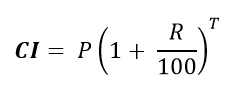## C program to calculate Compound Interest

Write a C program to input principle (amount), time and rate (P, T, R) and find Compound Interest. How to calculate compound interest in C programming. Logic to calculate compound interest in C program.

#### Required knowledge

Arithmetic operators, Operator precedence and associativity, Data types, Basic input/output

#### Compound Interest formula

Formula to calculate compound interest annually is given by.Where,

P is principle amount

R is the rate and

T is the time span

#### Logic to calculate compound interest

Step by step descriptive logic to find compound interest.

1. Input principle amount. Store it in some variable say principle.

2. Input time in some variable say time.

3. Input rate in some variable say rate.

4. Calculate compound interest using formula, CI = principle * pow((1 + rate / 100), time).

5. Finally, print the resultant value of CI.

Read more - Program to find power of a number

#### Program to calculate compound interest

```
/**
* C program to calculate Compound Interest
*/

#include <stdio.h>
#include <math.h>

int main()
{
float principle, rate, time, CI;

/* Input principle, time and rate */
printf("Enter principle (amount): ");
scanf("%f", &principle);

printf("Enter time: ");
scanf("%f", &time);

printf("Enter rate: ");
scanf("%f", &rate);

/* Calculate compound interest */
CI = principle* (pow((1 + rate / 100), time));

/* Print the resultant CI */
printf("Compound Interest = %f", CI);

return 0;
}
```

##### Output:
```
Enter principle (amount): 1200
Enter time: 2
Enter rate: 5.4
Compound Interest = 1333.099243
```
```
Recommended posts

Loop programming exercises index.
Basic programming exercises index.
If else programming exercises index.
Array programming exercises index.
String programming exercises index.
Pointer programming exercises index.
Star Patterns exercises index.
Number Patterns exercises index.
alphabet Patterns exercises index.
series program exercises index.

```